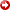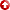[First Hit]Comparison of Total andEffectiveWater Saturations as a Way to Verify the Validity ofEffectivePorosity Calculations

Holmes, Michael, Antony Holmes, and Dominic Holmes
Digital Formation, Inc, Denver, CO

Ransom proposed that the following equality holds for standard oil and gas reservoirs.
In place hydrocarbons in total porosity = In place hydrocarbons ineffectiveporosity
Or:
ΦT*(1-SWT)=ΦE*(1-SWE)
Porosity * Hydrocarbons = Porosity * Hydrocarbons
Where:
ΦT = Total Porosity
SWT = Total Water Saturation
ΦE =EffectivePorosity
SWE =EffectiveWater Saturation
This relationship implies that there are no hydrocarbons in the shales.
Knowing total porosity, total water saturation andeffectiveporosity,effectivewater saturation can be calculated.Effectiveporosity is calculated from total porosity, shale volume, and porosity reading in shale.
ΦTE+VSHSH
Where:
VSH = Shale Volume
ΦSH = Shale Porosity
If either of the last two terms in inaccurate, the value ofeffectiveporosity will be inaccurate.
It can be shown that if either shale volume is overestimated and/ or shale porosity is overestimated, then calculations ofeffectiveporosity are too low. As a consequence, values of SWE are too low or even negative.
Examination of a large number of reservoirs shows that traditional choices of shale porosity often give negative values ofeffectivewater saturation. The incorrect choice of shale porosity will often not be obvious if only total water saturation is considered.
A plot of total vs.effectivewater saturation can help in the proper choice of shale porosity. A correct choice will result in data with similar values of shale volume aligned linearly, with different slopes converging to a single point where both total andeffectivewater saturation is one. An incorrect choice will lead to significant dispersion of the alignments.
Examples from a variety of reservoirs are included.

AAPG Search and Discovery Article #90071 © 2007 AAPG Rocky Mountain Meeting, Snowbird, Utah